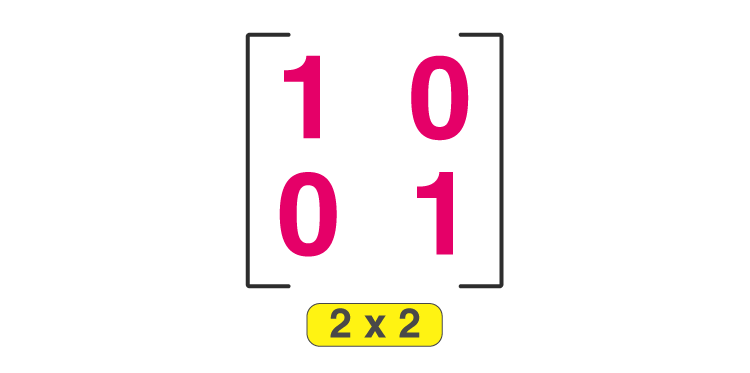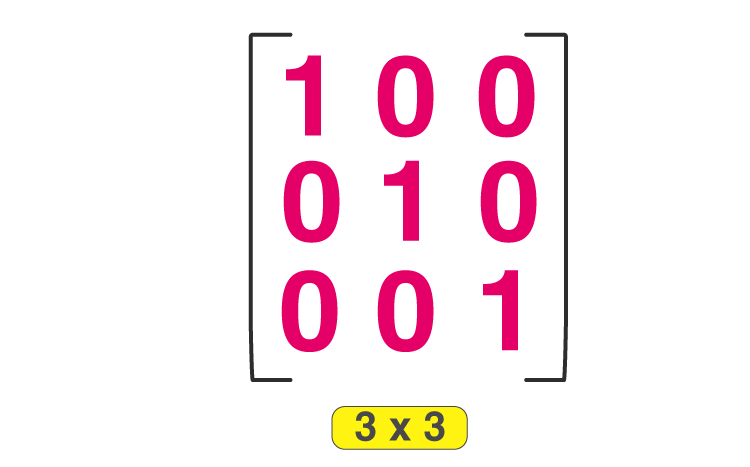# Identity Matrix

Identity Matrix is the matrix which is n × n square matrix where the diagonal consist of ones and the other elements are all zeros. It is also called as a Unit Matrix or Elementary matrix. It is represented as In or just by I, where n represents the size of the square matrix. For example,

$$\begin{array}{l}I_{1}=1\\I_{2}=\begin{bmatrix} 1 &0 \\ 0 &1 \end{bmatrix}\\ I_{3}=\begin{bmatrix} 1 & 0 & 0\\ 0 & 1 & 0 \\ 0 & 0 & 1 \end{bmatrix}\end{array}$$

We can also say, the identity matrix is a type of diagonal matrix, where the principal diagonal elements are ones, and rest elements are zeros. Let’s study about its definition, properties and practice some examples on it.

## Identity Matrix Definition

An identity matrix is a square matrix in which all the elements of principal diagonals are one, and all other elements are zeros. It is denoted by the notation “In” or simply “I”. If any matrix is multiplied with the identity matrix, the result will be given matrix. The elements of the given matrix remain unchanged. In other words,  if all the main diagonal of a square matrix are 1’s and rest all o’s, it is called an identity matrix. Here, the 2 × 2 and 3 × 3 identity matrix is given below:

2 × 2 Identity MatrixThis is also called the identity matrix of order 2.

3× 3 Identity MatrixThis is known as the identity matrix of order 3 or unit matrix of order 3 × 3.

Identity Matrix is donated by In × n, where n × n shows the order of the matrix.

A × I n × n = A, A = any square matrix of order n × n.

## Properties of Identity Matrix

1) It is always a Square Matrix

These Matrices are said to be square as it always has the same number of rows and columns. For any whole number n, there’s a corresponding Identity matrix, n × n.

2) By multiplying any matrix by the unit matrix, gives the matrix itself.

As the multiplication is not always defined, so the size of the matrix matters when we work on matrix multiplication.

Like, for “m × n” matrix C, we get

ImC = C = CIn

So the size of the matrix is important as multiplying by the unit is like doing it by 1 with numbers. For example:

$$\begin{array}{l}C = \begin{bmatrix} 1 & 2 & 3 &4 \\ 5& 6& 7 & 8 \end{bmatrix}\end{array}$$

The above is 2 × 4 matrix as it has 2 rows and 4 columns.

Let’s multiply the 2 × 2 identity matrix by C.

$$\begin{array}{l}\begin{bmatrix} 1 & 0\\ 0 & 1 \end{bmatrix}.\begin{bmatrix} 1 & 2 & 3 &4 \\ 5& 6& 7 & 8 \end{bmatrix}=\begin{bmatrix} 1+0 & 2+0 & 3+0 &4+0 \\ 0+5& 0+6& 0+7 & 0+8 \end{bmatrix} = \begin{bmatrix} 1 & 2 & 3 &4 \\ 5& 6& 7 & 8 \end{bmatrix}\end{array}$$

Hence proved.

3) We always get an identity after multiplying two inverse matrices.

If we multiply two matrices which are inverses of each other, then we get an identity matrix.

$$\begin{array}{l}C = \begin{bmatrix} 0 &1 \\ -2& 1 \end{bmatrix}\end{array}$$
$$\begin{array}{l}D=\begin{bmatrix} \frac{1}{2} &- \frac{1}{2} \\ 1& 0 \end{bmatrix}\end{array}$$
$$\begin{array}{l}CD= \begin{bmatrix} 0 &1 \\ -2& 1 \end{bmatrix}\begin{bmatrix} \frac{1}{2} &- \frac{1}{2} \\ 1& 0 \end{bmatrix}=\begin{bmatrix} 1 & 0\\ 0 & 1 \end{bmatrix}\end{array}$$
$$\begin{array}{l}DC = \begin{bmatrix} \frac{1}{2} &- \frac{1}{2} \\ 1& 0 \end{bmatrix}\begin{bmatrix} 0 &1 \\ -2& 1 \end{bmatrix}=\begin{bmatrix} 1 & 0\\ 0 & 1 \end{bmatrix}\end{array}$$

### Identity Matrix Examples

Example 1: Write an example of 4 × 4 order unit matrix.

Solution: The unit matrix is the one having ones on the main diagonal & other entries as ‘zeros’.

Thus, the unit matrix of order 4 × 4 or the identity matrix of order 4 can be written as:

$$\begin{array}{l}I_{4\times 4}=\begin{bmatrix} 1 & 0 & 0 &0 \\ 0& 1 & 0 &0 \\ 0 & 0 & 1 & 0\\ 0 & 0 & 0 &1 \end{bmatrix}\end{array}$$

Example 2: Check the following matrix is Identity matrix?

$$\begin{array}{l}V= \begin{bmatrix} 1 & 0 & 0 &0 \\ 0& 1 & 0 &0 \\ 0 & 0 & 1 & 0\\ \end{bmatrix}\end{array}$$

Solution: No, It’s not an identity matrix, because it is of the order 3 × 4, which is not a square matrix.

Example 3: Check the following matrix is Identity matrix;

$$\begin{array}{l}B =\begin{bmatrix} 1 & 1 & 1\\ 1 & 1& 1\\ 1 & 1 & 1 \end{bmatrix}\end{array}$$

Solution: No, it is not a unit matrix as it doesn’t contain the value of 0 beside one property of having diagonal values of 1.

Visit BYJU’S – The Learning App to explore a fun and interesting way to learn Mathematics.

## Frequently Asked Questions on Identity Matrix

Q1

### What do you mean by an identity matrix?

In linear algebra, an identity matrix is a matrix of order nxn such that each main diagonal element is equal to 1, and the remaining elements of the matrix are equal to 0.
Q2

### What is the identity matrix of a 2×2?

An identity matrix of 2×2 is a matrix with 1’s in the main diagonal and zeros everywhere. The identity matrix of order 2×2 is:
[1 0 0 1].
Q3

### What is the identity matrix of a 3×3?

An identity matrix of 3×3 is a matrix with 1’s in the main diagonal and zeros everywhere. The identity matrix of order 3×3 is given by:
[1 0 0 0 1 0 0 0 1].
Q4

### Is the identity matrix nonsingular?

Yes, the identity matrix is nonsingular since its determinant is not equal to 0. The identity matrix is the only idempotent matrix with a non-zero determinant. Therefore, we can also find the inverse of the identity matrix.
Q5

### How do you create an identity matrix?

As we know, the identity matrix has all its main diagonal elements as 1’s and the remaining elements 0’s. Suppose to create an identity matrix of order 4×4, we write the matrix elements in rows and columns as given below, and those should be enclosed within [ ].
1 0 0 0
0 1 0 0
0 0 1 0
0 0 0 1